## Correlation (Statistical)

For two variablesand,(1)

wheredenotes Standard Deviation and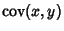is the Covariance of these two variables. For the general case of variables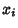and, where, 2, ...,,(2)

where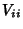are elements of the Covariance Matrix. In general, a correlation gives the strength of the relationship between variables. The variance of any quantity is alway Nonnegative by definition, so(3)

From a property of Variances, the sum can be expanded(4)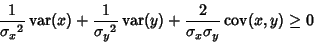(5)(6)

Therefore,(7)

Similarly,(8)(9)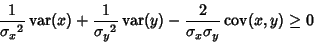(10)(11)

Therefore,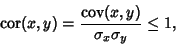(12)

so. For a linear combination of two variables,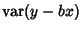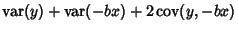(13)

Examine the cases where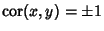,(14)(15)

The Variance will be zero if, which requires that the argument of the Variance is a constant. Therefore,, so. If,is either perfectly correlated (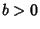) or perfectly anticorrelated (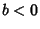) with.